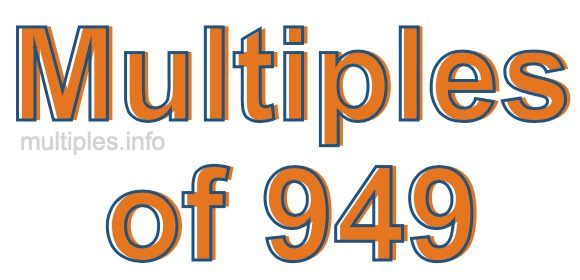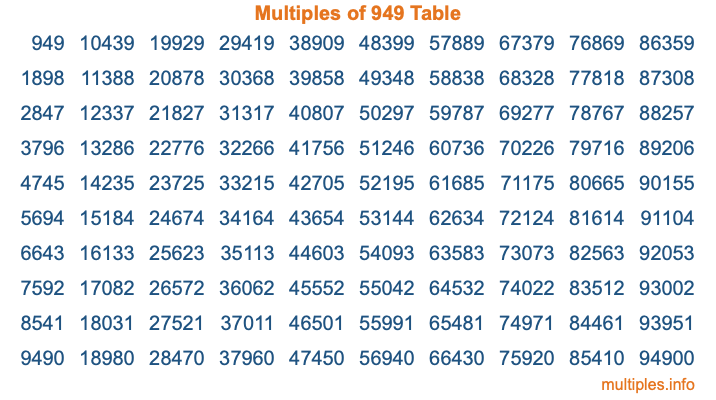Multiples of 949Welcome to the Multiples of 949 page. Here we will first teach you everything you will ever need to know about the multiples of 949, and then give you a study guide summary of everything we taught you to make sure you remember it all. Use this page to look up facts and learn information about the multiples of 949. This page will make you a multiples of nine hundred forty-nine expert!

Definition of Multiples of 949
Multiples of 949 are all the numbers that when divided by 949 equal an integer. Each of the multiples of 949 are called a multiple. A multiple of 949 is created by multiplying 949 by an integer.

Therefore, to create a list of multiples of 949, you start with 1 multiplied by 949, then 2 multiplied by 949, then 3 multiplied by 949, and so on for as long as you want. Thus, the list of the first five multiples of 949 is 949, 1898, 2847, 3796, and 4745. To see a larger list of multiples of 949, see the printable image of Multiples of 949 further down on this page. We also have a category where you can choose any nth multiple of 949.

Multiples of 949 Checker
The Multiples of 949 Checker below checks to see if any number of your choice is a multiple of 949. In other words, it checks to see if there is any number (integer) that when multiplied by 949 will equal your number. To do that, we divide your number by 949. If the the quotient is an integer, then your number is a multiple of 949.

Is  a multiple of 949?

Least Common Multiple of 949 and ...
A Least Common Multiple (LCM) is the lowest multiple that two or more numbers have in common. This is also called the smallest common multiple or lowest common multiple and is useful to know when you are adding our subtracting fractions. Enter one or more numbers below (949 is already entered) to find the LCM.

Check out our LCM Calculator if you need more details about the Least Common Multiple or if you need the LCM for different numbers for adding and subtraction fractions.

nth Multiple of 949
As we stated above, 949 is the first multiple of 949, 1898 is the second multiple of 949, 2847 is the third multiple of 949, and so on. Enter a number below to find the nth multiple of 949.

th multiple of 949

Multiples of 949 vs Factors of 949
949 is a multiple of 949 and a factor of 949, but that is where the similarities end. All postive multiples of 949 are 949 or greater than 949. All positive factors of 949 are 949 or less than 949.

Below is the beginning list of multiples of 949 and the factors of 949 so you can compare:

Multiples of 949: 949, 1898, 2847, 3796, 4745, etc.

Factors of 949: 1, 13, 73, 949

As you can see, the multiples of 949 are all the numbers that you can divide by 949 to get a whole number. The factors of 949, on the other hand, are all the whole numbers that you can multiply by another whole number to get 949.

It's also interesting to note that if a number (x) is a factor of 949, then 949 will also be a multiple of that number (x).

Multiples of 949 vs Divisors of 949
The divisors of 949 are all the integers that 949 can be divided by evenly. Below is a list of the divisors of 949.

Divisors of 949: 1, 13, 73, 949

The interesting thing to note here is that if you take any multiple of 949 and divide it by a divisor of 949, you will see that the quotient is an integer.

Multiples of 949 Table
Below is an image of the first 100 multiples of 949 in a table. The table is in chronological order, column by column. The first column has the first ten multiples of 949, the second column has the next ten multiples of 949, and so on.The Multiples of 949 Table is also referred to as the 949 Times Table or Times Table of 949. You are welcome to print out our table for your studies.

Negative Multiples of 949
Although not often discussed or needed in math, it is worth mentioning that you can make a list of negative multiples of 949 by multiplying 949 by -1, then by -2, then by -3, and so on, to get the following list of negative multiples of 949:

-949, -1898, -2847, -3796, -4745, etc.

Multiples of 949 Summary
Below is a summary of important Multiples of 949 facts that we have discussed on this page. To retain the knowledge on this page, we recommend that you read through the summary and explain to yourself or a study partner why they hold true.

There are an infinite number of multiples of 949.

A multiple of 949 divided by 949 will equal a whole number.

949 divided by a factor of 949 equals a divisor of 949.

The nth multiple of 949 is n times 949.

The largest factor of 949 is equal to the first positive multiple of 949.

949 is a multiple of every factor of 949.

949 is a multiple of 949.

A multiple of 949 divided by a divisor of 949 equals an integer.

949 divided by a divisor of 949 equals a factor of 949.

Any integer times 949 will equal a multiple of 949.

Multiples of a Number
Here you can get the multiples of another number, all with the same attention to detail as we did for multiples of 949 on this page.

Multiples of
Multiples of 950
Did you find our page about multiples of nine hundred forty-nine educational? Do you want more knowledge? Check out the multiples of the next number on our list!